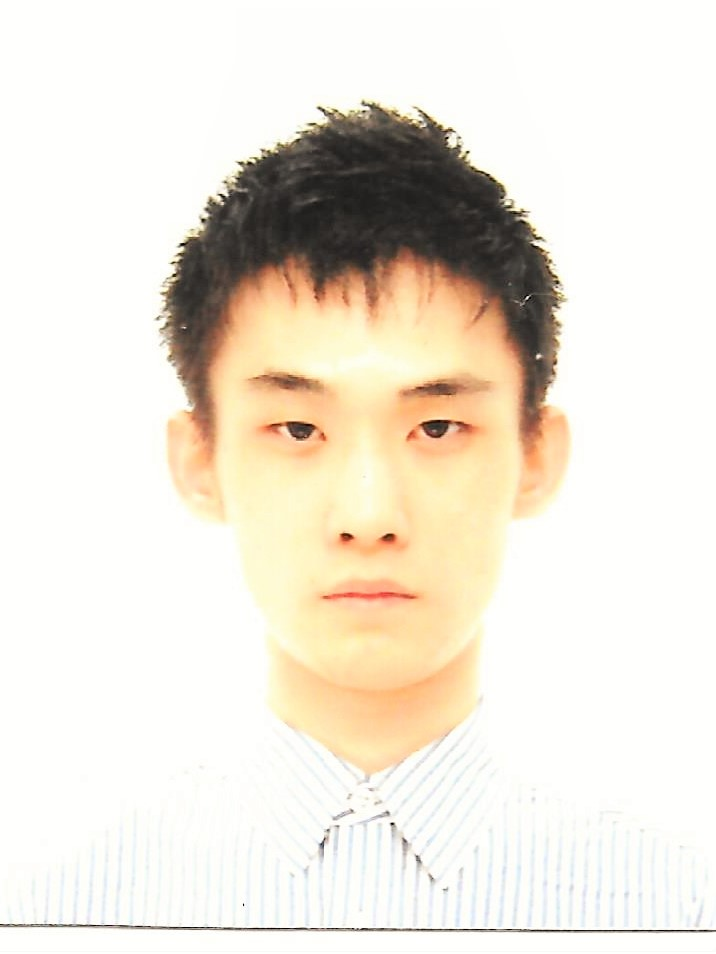## Course name

MathematicsAyahito Kira

Member of the Institute of Actuaries of Japan
FP Level 2
Statistics Test Level 2

Experience as a video course lecturer and tutor for the High School Entrance Examination

March 2018 – Graduated from the University of Tokyo, Department of Mathematical Informatics

Currently enrolled in Department of Mathematical Informatics, Graduate School of Information Science and Technology, The University of Tokyo

## Lecture schedule

The following lecture schedule is subject to change.

Lesson 1: Events and Probabilities, Conditional Probability, Bayes’ Theorem.

2 & 3: Random variables, probability density functions, distribution functions, expected value and variance, Chebyshev’s inequality, joint probability distributions, marginal probability distributions, conditional probability density, covariance, correlation coefficient.

4 & 5: Important distributions (binomial, Poisson, multinomial, hypergeometric, geometric, uniform, exponential, etc.).

6: Central Limit Theorem and normal distribution, normalization conversion, normal distribution table (z-scores).

7: Moment generating functions.

8: Probability density function after variable conversions (1 variable, 2 variables), determinant of Jacobi.

9: Population and sample, sample mean, sample variance, sample random variables.

10 & 11: Sample distribution in the normal population (normal distribution, chi-square distribution, F distribution, t distribution).

12: Point estimation, maximum likelihood estimation, optimality of the estimator (unbiased, validity, satisfiability, consistency).

13 & 14: Confidence Intervals and Significance Tests (including interval estimation and verification percentage in exponential distributions)

15: Regression analysis

16: Time series analysis

17: Stochastic processes

18: Simulations (random number generation)

19 & 20: Past Questions and Q & A

To those who wish to begin with review of high school and early university mathematics, we recommend attending the spring lecture course beginning April 14. Click here for details.

For the mathematics on the primary actuarial exam (probability, statistics, modeling), some people may be unfamiliar with the specific contents may not know how to proceed.  This course aims to teach probability and statistics from the very beginning, and systematically bring you up to the level necessary for passing the exam.  We will rely heavily on past released questions in preparation for the exam. (The ratio of lecture to exercises is scheduled to be around 60%: 40%)  For the exercises, we will primarily work on Question 1’s (basic).  However, when we get to Units 19 and 20, we will likely be working with Question 2’s and 3’s (intermediate and advanced).

We will proceed with lectures using the following teaching materials:

Main: Junichi Satsuma “Probability and Statistics (Introductory course for science and engineering mathematics 7)” Iwanami Shoten

Sub: Kiyoshi Kunisawa “Probability & Statistics Exercises 1 – Probability” and “Probability & Statistics Exercises 2 – Statistics” Baifukan

Practice Exercises: Past released problems

If you cannot attend class at any time, we are able to provide a video recording of the lecture or lectures missed for those who have paid full tuition at the time of application.  Please do not hesitate to take advantage of this service.## Questions・Inquiries

Email:  secretary@yoshidaand.co.jp
Phone:  080-4339-4650